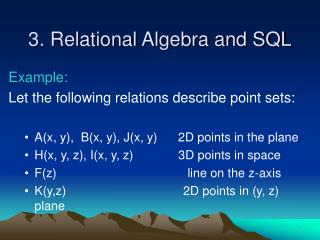DownloadDownload Presentation3. Relational Algebra and SQL

# 3. Relational Algebra and SQL

Download Presentation## 3. Relational Algebra and SQL

- - - - - - - - - - - - - - - - - - - - - - - - - - - E N D - - - - - - - - - - - - - - - - - - - - - - - - - - -
##### Presentation Transcript

1. 3. Relational Algebra and SQL Example: Let the following relations describe point sets: • A(x, y), B(x, y), J(x, y) 2D points in the plane • H(x, y, z), I(x, y, z) 3D points in space • F(z) line on the z-axis • K(y,z) 2D points in (y, z) plane

2. 3.1 Relation algebra operators A and B A  B A  B A \ B

3. A  F J K

4.  y,z H  x,y H  2x+z = 0 I

5. Example: Find the SSN and tax for each person. πSSN,Taxσwages+interest+capital_gain = income Taxrecord × Taxtable Example: Find the area of Lincoln reached by a radio station. ( πX,Y ( σName=“Lincoln” Town ) )  ( πX,Y Broadcast )

6. 3.2 SQL SELECT a1, ..., an FROM R1, R2, …, Rm WHERE Con1, …,Conk This means: πa1, ..., an( σ Con1( … (σ Conk ( R1× R2 × … ×Rm))…))

7. Example: Find the SSN and tax for each person. SELECT SSN, Tax FROM Taxrecord, Taxtable WHERE wages + interest + capital_gain = income

8. AS – keyword used to rename relations Two SQL expressions can be combined by: INTERSECT UNION MINUS– set difference

9. Example: Find the names of the streets that intersect. SELECT S.NAME, T.NAME FROM Streets AS S, Streets AS T WHERE S.X = T.X and S.Y = T.Y

10. Example: Assume we have the relations: Broadcast ( Radio, X , Y ) Town ( Name, X, Y ) Find the parts of Lincoln, NE that can be reached by at least one Radio station. (SELECT X, Y FROM Town WHERE Name = “Lincoln”) INTERSECT (SELECT X, Y FROM Broadcast)

11. Another way of connecting SQL expressions is using the IN keyword. SELECT …….. FROM …….. WHERE a IN ( SELECT b FROM ….. WHERE ….. )

12. SQL with aggregation – SELECT aggregate_function FROM ……. WHERE …… aggregate_function– Max (c1a1 + ……..+ cnan) where ai are attributes Min (c1a1 + ……..+ cnan) and ci are constants Sum(a) where a is an attribute that is Avg(a) constant in each constraint tuple Count(a)

13. Example: Package(Serial_No, From, Destination, Weight) Postage (Weight , Fee) Find the total postage of all packages sent from Omaha. SELECT Sum(Fee) FROM Package, Postage WHERE Package.Weight = Postage.Weight AND Package.From = “ Omaha “

14. GROUP BY– SELECT a1, …, an, aggregate_function FROM ….. WHERE …… GROUP BY a1, ..., ak • Evaluates basic SQL query • Groups the tuples according to different values of a1,..,ak • Applies the aggregate function to each group separately • {a1, …, ak}  {a1, …, an}

15. Example: Find the total postage sent out from each city. SELECT Package.From, Sum(Postage.Fee) FROM Package, Postage WHERE Package.Weight = Postage.Weight GROUP BY Package.From